# GSEB Solutions Class 12 Physics Chapter 8 Electromagnetic Waves

Gujarat Board GSEB Textbook Solutions Class 12 Physics Chapter 8 Electromagnetic Waves Textbook Questions and Answers, Additional Important Questions, Notes Pdf.

## Gujarat Board Textbook Solutions Class 12 Physics Chapter 8 Electromagnetic Waves

### GSEB Class 12 Physics Electromagnetic Waves Text Book Questions and Answers

Question 1.
Figure shows a capacitor made of two circular plates each of radius 12 cm and separated by 5.0 cm. The capacitor is being charged by an external source (not shown in the figure). The charging current is constant and equal to 0.15(a) a Calculate the capacitance and the rate of change of potential difference between the plates.
(b) Obtain the displacement current across the plates.
(c) Is Kirchhoff’s first rule (junction rule) valid at each plate of the capacitor?
Solution:
(a) r = 12 x 10-2m,
d = 5 x 10-3m,
I = 0.15 A
A = πr2 = π x (12 x 10-2)2m2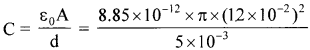= 80.1 x 10-12F = 80.1 pF
q = C V
I = $$\frac {dq}{dt}$$
C = $$\frac {dV}{dt}$$
$$\frac {dV}{dt}$$ = $$\frac {I}{C}$$ = $$\frac{0.15}{80.1 \times 10^{-12}}$$ = 1.875 x 109Vs-1
(b) Displacement current = Conduction current ID = 0.15 A
(c) YesQuestion 2.
A parallel plate capacitor (figure) made of circular plates each of radius R = 6.0 cm has a capacitance C = 100 pF. The capacitor is connected to a 230 V ac supply with a (angular) frequency of 300 rad s-1.
(a) What is the rms value of the conduction current?
(b) Is the conduction current equal to the displacement current?
(c) Determine the amplitude of B at a point 3.0 cm from the axis between the plates.
Solution:
R = 6.0 cm = 6 x 10-2m,
C = 100 pF = 100 x 10-12F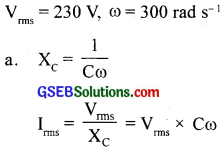= 230 x 100 x 10-12 x 300 = 6.9 x 10-6A = 6.9 μA

(b) Yes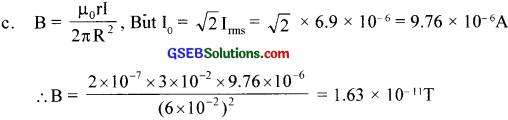Question 3.
What is the physical quantity the same for X-rays of wavelength 10-10 m, the red light of wavelength 6800 A, and radiowaves of wavelength 500 m?
Solution:
Both X-rays and red light are parts of the electromagnetic spectrum. They travel with the same speed in Vaccum

Question 4.
A plane electromagnetic wave travels in a vacuum along the z-direction. What can you say about the directions of its electric and magnetic field vectors? If the frequency of the wave is 30 MHz, what is its wavelength?
Solution:
$$\overrightarrow{\mathrm{E}}$$ and $$\overrightarrow{\mathrm{B}}$$ are in x – y plane and are mutually perpendicular,
v = υλ
λ = $$\frac {v}{υ}$$ = $$\frac{3 \times 10^{8}}{30 \times 10^{6}}$$ = $$\frac {3}{30}$$ x 102 = 10mQuestion 5.
A radio can tune into any station in the 7.5 MHz to 12 MHz hands. What is the corresponding wavelength band?
Solution:
υ1 = 7.5 MHz = 7.5 x 106 Hz.
υ2 = 12 x 106 Hz.
λ 1 = $$\frac{c}{v_{1}}$$ = $$\frac{3 \times 10^{8}}{7.5 \times 10^{6}}$$ = 40 m
λ 2 = $$\frac{c}{v_{2}}$$ = $$\frac{3 \times 10^{8}}{12 \times 10^{6}}$$ = 25 m
Wavelength band is 40 m – 25 m

Question 6.
A charged particle oscillates about its mean equilibrium position with a frequency of 109 Hz. What is the frequency of the electromagnetic waves produced by the oscillator?
Solution:
The frequency of the electromagnetic waves produced is the same as that of the oscillating charged particle. Hence the frequency of the electro­magnetic waves produced is, υ= 109 Hz.

Question 7.
The amplitude of the magnetic field part of a harmonic electromagnetic wave in a vacuum is B0 = 510 nT. What is the amplitude of the electric field part of the wave?
Solution:
B0 = 510 nT = 510 x 10-9T
E0 = ?
C = $$\frac{\mathrm{E}_{0}}{\mathrm{~B}_{0}}$$ ,
E0 = c
B0 = 3 x 108 x 510 x 10-9 = 153 NC-1

Question 8.
Suppose that the electric field amplitude of an electromagnetic wave is E0= 120 N/C and that its frequency is υ = 50.0 MHz.
(a) Determine, B0, ω, k. and . λ.
(b) Find the expressions for E and B
Solution:
E = 120 NC-1, u = 50 MHz = 50 x 106Hz
(a) B0 = $$\frac{\mathrm{E}_{0}}{\mathrm{c}}$$ = $$\frac{120}{3 \times 10^{8}}$$ = 4 x 10-7 T
ω = 2πω = 2 x 3 .14 x 50 x 10-6 = 3. 14 x 108 rad s-1
c = $$\frac{ω}{k}$$ ∴ k = $$\frac{ω}{c}$$ = $$\frac{3.14 \times 10^{8}}{3 \times 10^{8}}$$
λ = $$\frac{2π}{k}$$ = $$\frac{2 \times 3.14}{1.05}$$ = 5.98m

(b) E = E0sin(kx- ωt)= 120 sin(1.05x – 3.14 x 108t)Vm-1
B = B0 sin(kx – wt) = 4 x 10-7sin(1.05 x – 3.14 x 108t)TQuestion 9.
The terminology of different parts of the electromagnetic spectrum is given in the text. Use the formula E = hυ (for energy of a quantum of radiation: photon) and obtain the photon energy in units of eV for different parts of the electromagnetic spectrum. In what way are the different scales of photon energies that you obtain related to the sources of electromagnetic radiation?
Solution:
Photon energy (for λ = 1 m)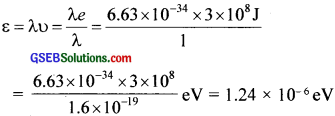Photon energy for other wavelengths in the figure for electromagnetic spectrum can be obtained by multiplying approximate powers often. Energy of a photon that a source produces indicates the spacings of the relevant energy levels of the source. For example, A = 10-12 m corresponds to photon energy = 1 .24 x 106 eV = 1.24 MeV. This indicates that nuclear energy levels (transition between which causes y -ray emission) ar typically spaced by 1 MeV or so. Similarly, a visible
wavelength = 5 x 10-7 m, corresponds to photon energy 2.5 eV. This implies that energy levels (transition between which gives visible radiation) are typically spaced by a few eV.

Question 10.
in a plane electromagnetic wave the electric field oscillates sinusoidally at a frequency of 2.0 x 1010 Hz and amplitude 48 V m-1.
(a) What is the wavelength of the wave?
(b) What is the amplitude of the oscillating magnetic field?
(c) Show that the average energy density of the E field equals the average energy density of the B field, [c = 3 x 108 ms-1]
Solution: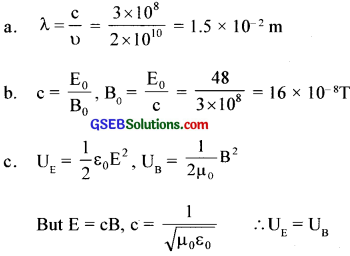Question 11.
Suppose that the electric field part of an electromagnetic wave in vacuum is
E = {(3.1 N/C) cos [(1.8 rad/m)y + (5.4 X 10’ rad/s)t]}$$\hat { i }$$.
(a) What is the direction of propagation?
(b) What is the wavelength )?
(c) What is the frequency?
d. What is the amplitude of the magnetic field part of the wave?
e. Write an expression for the magnetic field part of the wave.
Solution:
(a) – j

(b) We have E = {3. 14 N/C cos (1.8 rad/m)y + 5.4 x 106 radIs t}t
The standard equation is x = A sin [$$\frac {2π}{T}$$ t + $$\frac {2π}{λ}$$ y]
Comparing the above equation, $$\frac {2π}{λ}$$ y = 1.8 y
λ = $$\frac{2 \times 3.14}{1.8}$$ = 3.5 m

(c) We have $$\frac {2π}{T}$$ t = 5.4 x 106t
$$\frac {1}{T}$$ = $$\frac{5.4 \times 10^{6}}{2 \times 3.14}$$
υ = 0.859 x 106Hz

(d) c = $$\frac{E_{0}}{B_{0}}$$
B0 = $$\frac{E_{0}}{c}$$ = $$\frac{3.1}{3 \times 10^{8}}$$ = $$\frac{3.1 \times 10^{-8}}{3}$$ = 1.03 x 10-8 T

(e) B = {(1.03 x 10-8 T) cos (1.8 rad/m)y + (5.4 x 106rad/s)t}$$\hat {k}$$Question 12.
About 5% of the power of a loo W light bulb is converted to visible radiation. What is the average intensity of visible radiation
(a) at a distance of 1m from the bulb
(b) at a distance of 10 m
Assume that the radiation is emitted isotropically and neglect reflection.
Solution:
(a) A = 4πr2 = 4 x 3.14 x 12m2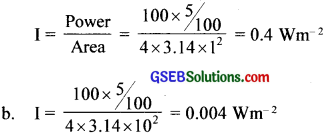Question 13.
Use the formula ? λmT = 0.29 cmK to obtain the characteristic temperature ranges for different parts of the electromagnetic spectrum. What do the numbers that you obtain tell you?
Solution:
A body at temperature T produces a continuous spectrum of wavelengths. For a black body. the wavelength corresponding to maximum intensity of radiation is given according to Planck’s law by the relation: λmT = 0.29 cm K. For λmT = 10-6 m. T = 2900 K. Using the same relation. temperatures for other wavelengths can be found. These numbers tell us the temperature ranges required for obtaining radiations in different parts of the electromagnetic spectrum. Thus, to obtain visible radiation. say = 5 x 10-7 m, the source should have a temperature of about 6000 K.
Note: a lower temperature will also produce this wavelength hut not of maximum intensity.

Question 14.
Given below are some famous numbers associated with electromagnetic radiations in different contexts in physics. State the part of the electromagnetic spectrum to which each belongs.
(a) 21 cm (wavelength emitted by atomic hydrogen in interstellar space).
(b) 1057 MHz (frequency of radiation arising from two close energy levels in hydrogen; known as Lamb shift).
(c) 2.7 K [temperature associated with the isotropic radiation filling all space-thought to be a relic of the big-bang’ origin of the universe].
(d) 5890 Å – 5896 Å [double lines of sodium]
(e) 14.4 keV [energy of a particular transition in 57Fe nucleus associated with a famous
high resolution spectroscopic method (Mössbauer spectroscopy)].
Solution:
(a) Radiation of wavelength of 21 cm:- It corresponds to radio waves of short wavelength.

(b) Radiation of frequency of 1,057 MHz: It also corresponds to radio waves of short oil. wavelength [or of high frequency].

(c) Temperature of 2.7 K: As obtained in the above question this temperature corresponds to the emission of microwaves.

(d) Double lines of sodium [5,890 A – 5,869 A]: These lines correspond to visible light in yellow. region.

(e) Energy of 14.4 ke V: As obtained in the question above, this energy corresponds to X-rays of low energy (Or soft – X-rays),Question 15.
(b) It is necessary to use satellites for long-distance TV transmission. Why?
(c) Optical and radio telescopes are built on the ground, but X-ray astronomy is possible only from satellites orbiting the earth. Why?
(d) The small ozone layer on top of the stratosphere is crucial for human survival. Why?
(e) If the earth did not have an atmosphere, would its average surface temperature be higher or lower than what it is now?
(f) Some scientists have predicted that a global nuclear war on the earth would be followed by a severe ‘nuclear winter’ with a devastating effect on life on earth. What might be the basis of this prediction?
Solution:
(a) The shortwaves (wavelength less than 200m or frequencies greater than 1,500 kHz) are absorbed by the earth due to their high frequency but are effectively reflected by F­layer in the ionosphere. After reflection from the ionosphere, the short waves reach the surface of the earth back only at a large distance from the transmitter. For this reason, short waves are used in long-distance transmission.

(b) Television signals are not reflected by the ionosphere. The TV signals from an earth station are reflected back to the earth by making use of artificial satellites.

(c) The earth’s atmosphere is transparent to visible light and radio waves but absorbs X-rays. Therefore, X-ray astronomy is possible only from the satellites orbiting the earth.

(d) The ultraviolet radiation from the sun is harmful to live cells and plants. The ozone layer absorbs ultra-violet radiation and prevents it from reaching the earth. It also keeps the earth warm by trapping infrared radiation.

(e) The infra-red radiation emitted by the earth is retained by the earth’s atmosphere due to the greenhouse effect and this keeps the earth warm. If the earth didn’t have an atmosphere, its average temperature would have been low.

(f) Scientists estimate that in case of a global nuclear war, the clouds produced will cover probably the whole of the sky. In that case, solar radiation would be prevented from reaching the earth and it will result in, what they call nuclear winter on the earth.

### GSEB Class 12 Physics Electromagnetic Waves Additional Important Questions and Answers

Question 1.
What is an electromagnetic wave?
Electromagnetic waves are periodically changing electric and magnetic fields, which propagate through space. The electric and magnetic field vectors are at right angles to each other and at right angles to the direction of propagation.

Question 2.
What are the contributions of Faraday and Maxwell in this field?
Faraday – Varying magnetic field produces an electric field.
Maxwell – Varying electric field produces magnetic Field

Question 3.
Match the following.

 A B C i. y ray Sunburn Photon emission by fast-moving electrons ii. X-ray Remote sensing Electronic de-excitation iii. UV ray Diagnosis Oscillating current iv. Microwave Radioactivity Nucleus

 A B C i. y ray Radioactivity Nucleus ii. X-ray Diagnosis Photon emission by fast-moving electrons iii. UV ray Sunburn Electronic de-excitation iv. Microwave Remote sensing Oscillating current

Question 4.
(a) If the fundamental source of a sound wave is a vibrating object. what is the fundamental source of an electromagnetic wave?
(b) Give the expression for the velocity of E.M waves in a vacuum.
(c) On what factors does its velocity in vacuum depend?
(a) The varying electric and magnetic fields can act as sources of each other and electromagnetic wave is produced.
(b) Velocity = c = $$\frac{1}{\sqrt{\mu_{0} \varepsilon_{0}}}$$
(c) Permeability (µ0) and permittivity (ε0) of free space

Question 5.
Maxwell noticed that Ampere’s circuital law is inconsistent where the electric current changes with time. He showed that consistency requires an additional source of the magnetic field.
(a) What is the current called and which is responsible for the magnetic field?
(b) Give the expression for this type of current.
(c) How it differs from conduction current?
(a) Displacement current is a time-varying electric field.
(b) Displacement current I = εA $$\frac { dE }{ dt }$$
(c) Displacement current is a time-varying electric field while conduction current is due to the flow of electrons in the circuit.
Displacement current does not exist under steady conditions, while conduction current exists even if the flow of electrons is at a uniform rate.Question 6.
(a) What is an electromagnetic spectrum?
(b) Flow the electromagnetic waves are arranged?
(c) Mention some of the properties of E.M waves.
(a) Electromagnetic waves cover a wide range of frequencies or wavelengths. An orderly arrangement of electromagnetic radiations from gamma rays of very short wavelength to radio waves of long wavelength is known as the electromagnetic spectrum.

(b) In the order of increasing wavelength, they are arranged as y – rays, X – rays, U.V. rays, visible light, infrared radiations, microwaves, and radio waves.

(c) i. Produced by accelerated charges.
ii. Do not require any material medium for propagation.
iii. Velocity of electromagnetic waves in free space is equal to that of light in free space.
iv. Obey the principle of superposition.
v. They carry energy as they propagate through space.

Question 7.
The electric field vector of an electromagnetic wave is E = E0 sin [2π($$\frac {x}{λ}$$ – $$\frac { t}{T}$$)]$$\hat {j}$$
(a) Write the magnetic field vector of this wave.
(b) What is the direction of propagation?
(c) With what velocity ¡he wave propagates?
(a) $$\bar { B }$$ = B0 sin [2π($$\frac {x}{λ}$$ – $$\frac { t}{T}$$)]$$\hat {k}$$
(b) x – direction (or along $$\hat {i}$$)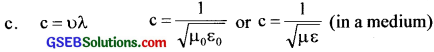Question 8.
(a) The small ozone layer on the top of the stratosphere is crucial for human survival Why?
(b) Where is the position of UV radiation in the electromagnetic spectrum?
(c) Can you convert UV to visible radiation? Explain.
(d) Which radiation is adjacent to UV to the higher frequency side of the electromagnetic spectrum?
(a) Ozone layer absorbs UV radiation which is harmful to humans.
(b) Between X – rays and visible light.
(c) Yes. In mercury tube light, [V is converted to visible radiation]
(d) X – rays

Question 9.
Both radio waves and gamma rays are transverse in nature and moving at the same speed in free space. Then in what aspect are they different?
i. Radiowaves have an atomic origin while gamma rays have a nuclear origin.
ii. Penetrating power of radiowaves is small while that of gamma rays is large.

Question 10.
Prof. J. Prakash asked Viji whether the average energy density of the electric field and magnetic field are equal or not? Vìji said “yes“. Justify the answer.
Velocity,c = $$\frac{1}{\sqrt{\mu_{0} \varepsilon_{0}}}$$ and B0 = $$\frac{\mathrm{E}_{0}}{\mathrm{c}}$$
The amplitude of oscillating electric field = E0
The amplitude of oscillating magnetic field = B0
Average energy density in E field = $$\frac {1}{2}$$ ε0E0
Average energy density in B field = $$\frac{1}{2 \mu_{0}}$$ $$\mathrm{B}_{0}^{2}$$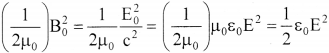∴VB = VE
Hence the proofQuestion 11.
What is the greenhouse effect? Explain.
The earth’s surface absorbs solar radiation and gets heated up. Then like an other hot body, it starts emitting radia tions. The radiations emitted by the earth are mainly infrared radiations. These radiations are unable to cross the lower atmosphere. which reflects them back. Low lying clouds also prevent IR radiation from passing through and thus serve to keep the earth’s surface warm at night. This phenomenon is called the Greenhouse Effect’.Question 12.
Radio waves diffract around the building, while light waves even though electromagnetic do not. Win’? (IISE M 99)
Radiowaves have wavelength greater than 10m while that of light is of the order of 10-10m Hence the reason.

Question 13.
Why do we use short waves for bug-distance radio broadcasts? (HSE say 2002)
The short radio waves are easily reflected by the ionosphere.

Question 14.
Why are microwaves used in RADAR?